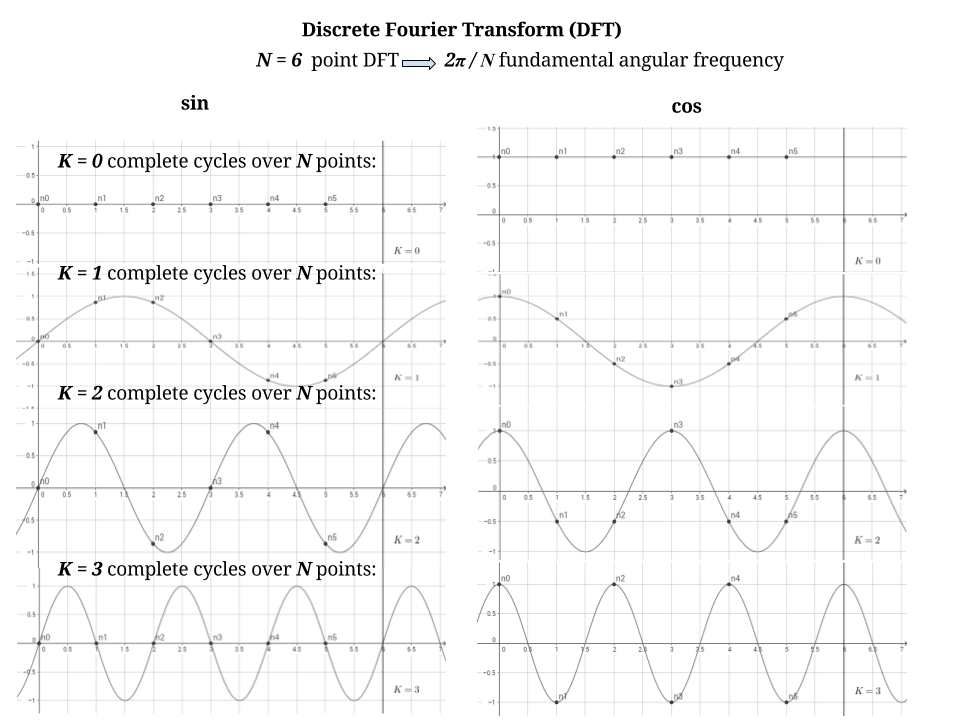### DISCRETE FOURIER TRANSFORMATION:

This is a great reference.

We are dealing with $$N=6$$ points, corresponding to integers from $$0$$ to $$N-1=5$$, and the sinusoids are truncated at length $$N$$. There are $$N$$ basis functions of amplitude $$1$$: $$N/2$$ sine, and $$N/2$$ cosine according to The Scientist and Engineer’s Guide to Digital Signal Processing by Steven W. Smith, and I’m trying to get the explanation for it here.

The fundamental angular frequency is given by $$\frac{2\pi}{N}$$, which can be thought of as slicing the $$2\pi$$ circle into $$N$$ equal slices.

The frequency of each sinusoid is determined by the parameter $$k$$. The frequency parameter, $$k$$, is equal to the number of complete cycles that occur over the $$N$$ points of the signal.

Each point on the left column of the plot below is calculated as $$\sin\left(\frac{2\pi}{N}kn\right)$$ with $$n\in\{0,\dots,5\}$$. The first plot is for $$k=0$$; the second, $$k=1$$; $$k=2$$ and, finally, $$k=3$$. The column on the right is calculated as $$\cos\left(\frac{2\pi}{N}kn\right)$$.The dots in the plots above were simply defined as $$(x,f(x))=(0,f(0)), (1,f(1)), (2,f(2)),(3,f(3)),(4,f(4)),(5,f(5))$$ with $$f(x)=\exp\{\frac{2\pi}{N}kn\}=\exp\{\frac{2\pi}{6}kx\}$$. The amplitude is $$1$$. $$k$$ was advanced from $$0$$ to $$N/2=3$$ for both sine and cosine basis functions.

The DFT is defined as:

$X(k)\equiv \sum_{n=0}^{N-1}\underset{\text{time sequence}}{\underbrace{ x(n)}}\; \underset{\text{basis funcs.}}{\underbrace{\exp\left(\color{red}{\mathbf-}i\frac{2\pi}{N}kn\right)}}, \quad k=0,1,\dots,N-1$

although also found with a normalizing $$1/N$$ in front:

$X(k)\equiv \frac{1}{N}\sum_{n=0}^{N-1}\underset{\text{time sequence}}{\underbrace{ x(n)}}\; \underset{\text{basis funcs.}}{\underbrace{\exp\left(\color{red}{\mathbf-}i\frac{2\pi}{N}kn\right)}}, \quad k=0,1,\dots,N-1$

The minus sign is just a convention so that the inverse (IDFT) ends up expressed as the sum of positive frequencies:

$x[n]= \sum_k X(k) \exp\left(i\,\frac{2\pi}{N} kn\right)$

which is a sum of sines and cosines if we use the Euler’s formula:

$e^{ix}=\cos x + i \sin x$There is another explanation of this convention here. The only important part is that the direct and inverse DFT have opposite signs.

We definine $$w_N$$ - the twiddle factor:

$\large w_N \equiv e^{-i\,\frac{2\pi}{N}}$

which has periodic properties. The $$N$$ power of the twiddle factor is:

$w_N^N=e^{-i\,\frac{2\pi}{N}N}=e^{-i2\pi}=1$

thanks to Euler’s identity, $$e^{i\pi}+1 = 0$$, and $$e^{-i2\pi}=1$$.

And $$w_N^{k+N}=w_N^k$$ because $$w_N^{k+N}=w_N^k\,w_N^N$$.

The exponents are $$w_N^{kn}=\left(e^{-i\,\frac{2\pi}{N}}\right)^{kn}$$.

It makes sense then to express the DFT as a matrix. The $$N=4$$ (four-point) DFT is especially cool, because splitting the circle into quadrant result in pure sine and cosine waves - in the complex plane, we are either along the $$x$$-axis (real / cosine), or along the $$y$$-axis (imaginary /sine). The matrix (really well explained by Professor Stang) then simplifies nicely as:

$W = \begin{bmatrix} \omega^0 & \omega^0 &\omega^0 &\omega^0 \\ \omega^0 & \omega^1 &\omega^2 &\omega^3 \\ \omega^0 & \omega^2 &\omega^4 &\omega^6 \\ \omega^0 & \omega^3 &\omega^6 &\omega^9 \\ \end{bmatrix} = \begin{bmatrix} 1 & 1 & 1 & 1\\ 1 & -i & -1 & i\\ 1 & -1 & 1 & -1\\ 1 & i & -1 & -i\end{bmatrix}$

where $$\omega = e^{-\frac{\pi i}{2}} = -i.$$

The DFT would therefore be calculated as:

$\begin{bmatrix} X\\X\\X\\X \end{bmatrix}= \begin{bmatrix} \omega^0 & \omega^0 &\omega^0 &\omega^0 \\ \omega^0 & \omega^1 &\omega^2 &\omega^3 \\ \omega^0 & \omega^2 &\omega^4 &\omega^6 \\ \omega^0 & \omega^3 &\omega^6 &\omega^9 \\ \end{bmatrix} \begin{bmatrix} x\\x\\x\\x \end{bmatrix}$

leading to the Fast Fourier (FFT) expedited method.

There is a great insight found here:

Defining $$s_k(n)\equiv e^{i\,\frac{2\pi}{N}kn}$$.

Finally, we need to understand what the summation over $$n$$ is doing in the definition of the DFT. We’ll learn that it should be seen as the computation of the inner product of the signals $$x$$ and $$s_k$$ defined above, so that we may write the DFT, using inner-product notation, as

$X(k) \equiv \langle x,s_k\rangle$

where $$s_k(n) \equiv e^{j{2\pi n k/N}}$$ is the sampled complex sinusoid at (normalized) radian frequency $$\omega_k T=2\pi k/N$$, where $$T$$ is the sampling interval or sampling period, and the inner product operation $$\left<\,\cdot\,,\,\cdot\,\right>$$ is defined by $$\left<x,y\right> \equiv \displaystyle\sum_{n=0}^{N-1}x(n) \overline{y(n)}$$.

This idea of DFT as a measure of similarity between the sampled signal and the sinusoids is very well explained on this video, containing a great example: